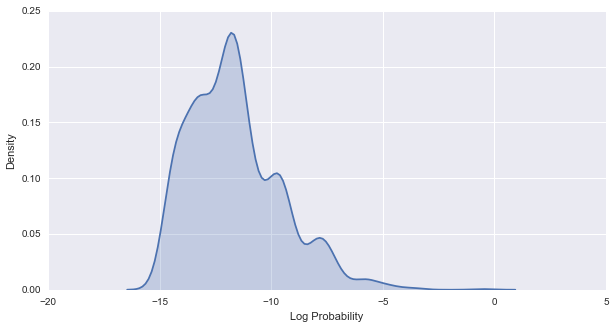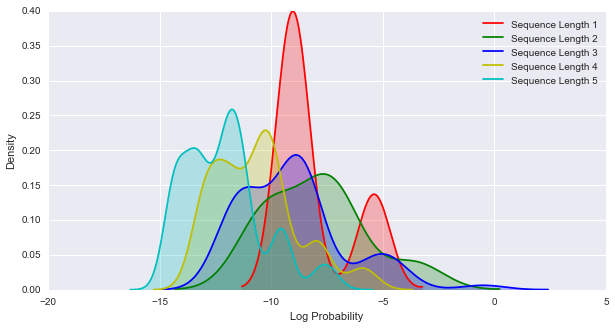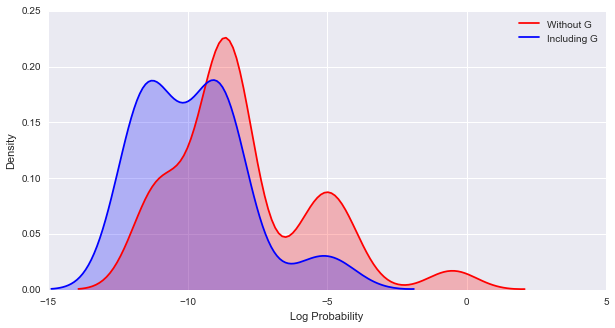This example is taken from the YAHMM wiki on silent states and edited.

# Global Sequence Alignment using YAHMM¶

Global sequence alignment is a problem in bioinformatics which involves aligning two sequences against each other in the maximally likely way. Lets say that you have the two sequences, ACT and ACG, and you wish to align them. The most obvious alignment is:

ACT
||
ACG

It's a close match, but one sequence has a G and one has a T at the end. You know from biology that nucleotides can mutate over time, be removed, or be added. In this case, you have to weigh the two hypotheses of 'did this nucleotide mutate' vs 'was a nucleotide removed and then another one added later'. Almost always the nucleotide mutation hypothesis wins out.###### Picture from Biological Sequence Analysis: Probabilistic Methods of Proteins and Nucleic Acids by Durbin, Eddy, Krogh, and Mitchison

This alignment can be done through the use of a profile HMM, pictured above. It contains matches along the bottom, inserts in the middle, and deletes along the top. The deletes are silent states, which are almost always pictured as circles in cartoons. In this example, the profile has M1='A', M2='C', and M3='T', with some pseudocounts for the other possibilities, and the sequence we wish to align is 'ACG'. The most likely path would be to follow the match states through, even though the 'G' does not align to the 'T' at M3.

Lets look at another sequence alignment.

ACGTTGCGACGACAAAACT
||||| |          ||
ACGTTCC----------CT

The bottom sequence seems to be missing a chunk. This is a common phenomena, and can be related to a protein losing an entire domain over a long stretch of time for some evolutionary reason. If the top sequence was the profile, and the bottom sequence was being aligned to the model, we couldn't take the match states through. After reaching the aligning the seventh nucleotide ('C') to a match state, there's a long stretch to get to the next match state.

This is where silent states play a role. You don't need to emit a character to travel along them, though you do have to suffer the transition probability cost. We can skip from what would be M7 to D8, all the way down to D17, then transition back to M18 to align to the 'C' and 'T' at the end of the model, all without emitting a character while going along delete states. This is an extremely useful part of hidden Markov models, and not all packages contain them.

We can see that the deletes allow for transitions from the beginning to the very end, without having to align to more than one character generating state, the first insert state. This allows the profile in the HMM to have sequences of variable length align to it, without cluttering the model with edges from every insert and match to every future insert and match. Lets create this very example.

Insertions will be modelled as symbol-emitting states with uniform probabilities for each nucleotide. We believe that the probability of an insertion into the actual sequence does not depend on the position, and so the underlying distribution behind each of these states should be tied. This means that when these states are trained, they are all considered a single distribution, and will all have the same distribution post-training.

Doing this by hand is a lot of edges and transitions. Typically, you can use a loop to build a model like this. An example is at https://github.com/jmschrei/PyPore/blob/master/alignment.py, under ProfileAligner._build_global(), which builds a global alignment HMM for Gaussian emissions, but can easily be changed to represent discrete characters by changing the GaussianKernelDensity distribution to DiscreteDistribution.

Now, lets build this model using YAHMM. We start off by creating the Model instance.

In :
from yahmm import *
model = Model( name="Global Sequence Aligner" )


The graphical structure of the model is now defined node by node, and edge by edge. This allows for complicated structures to be made easily, even if they do require a few more lines of code.

In :
# Define the distribution for insertions
i_d = DiscreteDistribution( { 'A': 0.25, 'C': 0.25, 'G': 0.25, 'T': 0.25 } )

# Create the insert states
i0 = State( i_d, name="I0" )
i1 = State( i_d, name="I1" )
i2 = State( i_d, name="I2" )
i3 = State( i_d, name="I3" )

# Create the match states
m1 = State( DiscreteDistribution({ "A": 0.95, 'C': 0.01, 'G': 0.01, 'T': 0.02 }) , name="M1" )
m2 = State( DiscreteDistribution({ "A": 0.003, 'C': 0.99, 'G': 0.003, 'T': 0.004 }) , name="M2" )
m3 = State( DiscreteDistribution({ "A": 0.01, 'C': 0.01, 'G': 0.01, 'T': 0.97 }) , name="M3" )

# Create the delete states
d1 = State( None, name="D1" )
d2 = State( None, name="D2" )
d3 = State( None, name="D3" )

# Add all the states to the model
model.add_states( [i0, i1, i2, i3, m1, m2, m3, d1, d2, d3 ] )

# Create transitions from match states

# Create transitions from insert states

# Create transitions from delete states

# Call bake to finalize the structure of the model.
model.bake()


The profile created will be of the 3 nucleotide sequence 'ACT', with pseudocounts at each position to allow for the possibility of small deviations. Lets calculate the viterbi path across a few small sequences.

In :
for sequence in map( list, ('ACT', 'GGC', 'GAT', 'ACC') ):
logp, path = model.viterbi( sequence )
print "Sequence: '{}'  -- Log Probability: {} -- Path: {}".format(
''.join( sequence ), logp, " ".join( state.name for idx, state in path[1:-1] ) )

Sequence: 'ACT'  -- Log Probability: -0.513244900357 -- Path: M1 M2 M3
Sequence: 'GGC'  -- Log Probability: -11.0481012413 -- Path: I0 I0 D1 M2 D3
Sequence: 'GAT'  -- Log Probability: -9.12551967402 -- Path: I0 M1 D2 M3
Sequence: 'ACC'  -- Log Probability: -5.08795587886 -- Path: M1 M2 M3


The first and last sequence are entirely matches, meaning that it thinks the most likely alignment between the profile ACT and ACT is A-A, C-C, and T-T, which makes sense, and the most likely alignment between ACT and ACC is A-A, C-C, and T-C, which includes a mismatch. Essentially, it's more likely that there's a T-C mismatch at the end then that there was a deletion of a T at the end of the sequence, and a separate insertion of a C.

The two middle sequences don't match very well, as expected! G's are not very likely in this profile at all. It predicts that the two G's are inserts, and that the C matches the C in the profile, before hitting the delete state because it can't emit a T. The third sequence thinks that the G is an insert, as expected, and then aligns the A and T in the sequence to the A and T in the master sequence, missing the middle C in the profile.

By using deletes, we can handle other sequences which are shorter than three characters. Lets look at some more sequences of different lengths.

In :
for sequence in map( list, ('A', 'GA', 'AC', 'AT', 'ATCC', 'ACGTG', 'ATTT', 'TACCCTC', 'TGTCAACACT') ):
logp, path = model.viterbi( sequence )
print "Sequence: '{}'  -- Log Probability: {} -- Path: {}".format(
''.join( sequence ), logp, " ".join( state.name for idx, state in path[1:-1] ) )

Sequence: 'A'  -- Log Probability: -5.40618101242 -- Path: M1 D2 D3
Sequence: 'GA'  -- Log Probability: -10.8868199358 -- Path: I0 M1 D2 D3
Sequence: 'AC'  -- Log Probability: -3.62447187905 -- Path: M1 M2 D3
Sequence: 'AT'  -- Log Probability: -3.64488075068 -- Path: M1 D2 M3
Sequence: 'ATCC'  -- Log Probability: -10.6743329646 -- Path: M1 D2 M3 I3 I3
Sequence: 'ACGTG'  -- Log Probability: -10.3938248352 -- Path: M1 M2 I2 I2 I2 D3
Sequence: 'ATTT'  -- Log Probability: -8.67126440175 -- Path: M1 I1 I1 D2 M3
Sequence: 'TACCCTC'  -- Log Probability: -16.9034517961 -- Path: I0 I0 I0 I0 D1 M2 M3 I3
Sequence: 'TGTCAACACT'  -- Log Probability: -16.4516996541 -- Path: I0 I0 I0 I0 I0 I0 I0 M1 M2 M3


Again, more of the same expected. You'll notice most of the use of insertion states are at I0, because most of the insertions are at the beginning of the sequence. It's more probable to simply stay in I0 at the beginning instead of go from I0 to D1 to I1, or going to another insert state along there. You'll see other insert states used when insertions occur in other places in the sequence, like 'ATTT' and 'ACGTG'.

Now that we have the path, we need to convert it into an alignment, which is significantly more informative to look at.

In :
def path_to_alignment( x, y, path ):
"""
This function will take in two sequences, and the ML path which is their alignment,
and insert dashes appropriately to make them appear aligned. This consists only of
adding a dash to the model sequence for every insert in the path appropriately, and
a dash in the observed sequence for every delete in the path appropriately.
"""

for i, (index, state) in enumerate( path[1:-1] ):
name = state.name

if name.startswith( 'D' ):
y = y[:i] + '-' + y[i:]
elif name.startswith( 'I' ):
x = x[:i] + '-' + x[i:]

return x, y

for sequence in map( list, ('A', 'GA', 'AC', 'AT', 'ATCC' ) ):
logp, path = model.viterbi( sequence )
x, y = path_to_alignment( 'ACT', ''.join(sequence), path )

print "Sequence: {}, Log Probability: {}".format( ''.join(sequence), logp )
print "{}\n{}".format( x, y )
print

Sequence: A, Log Probability: -5.40618101242
ACT
A--

Sequence: GA, Log Probability: -10.8868199358
-ACT
GA--

Sequence: AC, Log Probability: -3.62447187905
ACT
AC-

Sequence: AT, Log Probability: -3.64488075068
ACT
A-T

Sequence: ATCC, Log Probability: -10.6743329646
ACT--
A-TCC



In addition to getting this alignment, we can do some interesting things with this model! Lets score every sequence of length 5 of less and see what the distribution looks like.

In :
import itertools

sequences = reduce( lambda x, y: x+y, [[ seq for seq in it.product( 'ACGT', repeat=i ) ] for i in xrange( 1,6 )] )
scores = map( model.log_probability, sequences )


Now lets plot a kernel density of that distribution.

In :
import seaborn as sns
%pylab inline

plt.figure( figsize=(10,5) )
sns.kdeplot( numpy.array( scores ), shade=True )
plt.ylabel('Density')
plt.xlabel('Log Probability')
plt.show()

Populating the interactive namespace from numpy and matplotlib

WARNING: pylab import has clobbered these variables: ['log', 'i0', 'random', 'exp']
%matplotlib prevents importing * from pylab and numpyThere seem to be five local maxima. We can interrogate if these correspond to each of the sequence lengths by just plotting them out individually.

In :
plt.figure( figsize=(10,5) )
for i in xrange( 1, 6 ):
subset = it.product( 'ACGT', repeat=i )
subset_scores = map( model.log_probability, subset )
sns.kdeplot( numpy.array( subset_scores ), color='rgbyc'[i-1], shade=True, label="Sequence Length " + str(i) )
plt.ylabel('Density')
plt.xlabel('Log Probability')
plt.legend()

Out:
<matplotlib.legend.Legend at 0x17196d30>Well, that hypothesis was incorrect! We can say that the red peak just above a log score of -10 is likely associated with the same peak in the previous graph, but the others are likely more complicated. One last thing we can do is look at the distribution of sequences which do not contain 'G' compared to those which do, because 'G' does not appear in the profile at all.

In :
plt.figure( figsize=(10, 5) )

subsequences = it.product( 'ACT', repeat=3 )
scores = map( model.log_probability, subsequences )

sns.kdeplot( numpy.array( scores ), color='r', shade=True, label='Without G' )

subsequences = filter( lambda seq: 'G' in seq, it.product( 'ACGT', repeat=3 ) )
scores = map( model.log_probability, subsequences )

sns.kdeplot( numpy.array( scores ), color='b', shade=True, label='Including G')

plt.ylabel('Density')
plt.xlabel('Log Probability')
plt.legend()

Out:
<matplotlib.legend.Legend at 0x17681cf8>Well, that's mildly informative. We see that sequences which contain 'G' in them are on average less likely, but still pretty close to sequences which do not contain 'G'. You'll notice in the code we filtered out any sequence which did not contain 'G' from the second group, to ensure all sequences scored contained at least one 'G' in them.

## YAHMM Information¶

YAHMM is freely available under the MIT license at https://github.com/jmschrei/yahmm. Feel free to contribute or comment on the library!

Installing YAHMM is easy, but simply calling pip install yahmm. Dependencies are numpy, scipy, matplotlib, networkx, and Cython. If you do not have these, and pip fails when building the prerequisites, the Anaconda distribution is a good way to get many scientific packages for Python, and has been shown on multiple platforms to allow YAHMM to install.

If you have questions or comments, my email is [email protected] Please let me know how you use YAHMM to solve your problems!

In :